# Random Walk Simulation Of Stock Prices Using Geometric Brownian Motion

In this blog on random walk simulation, we will learn how to simulate stock prices. Future stock prices are very hard to predict and are dependent on the past trend and volatility. While simulating the stock prices one has to give reasonable weightage to these two parameters. The random walk model helps incorporate these two features of a stock and simulate the stock prices in a very clear and simple way. A random walk is a mathematical object, known as a stochastic or random process, that describes a path that consists of a succession of random steps on some mathematical space. Needless to say, the assumption that stock prices are random and cannot be predicted is at the core of this model. Let us first understand the mathematical equation that forms the basis of our simulation:

`Xt = μ + Xt-1+ et`

Where, Xt is the log of stock price at time t Xt-1 is the log of stock price at time t-1 μ is the drift constant eis the white noise at time t

### Geometric Brownian Motion (GBM):

As you can see, except μ all the other terms change with time and the random noise will change at every time step. In a standard random walk, the model takes steps of size one at every integer time point and has an equal chance to go up or down. In the above formula, we have chosen a variable step size at every time step. If we take steps of size √h at times which are multiples of h and then take the limit as h→0, you get a Brownian motion. If we look at the definition of a Geometric Brownian Motion it states that:

"A Geometric Brownian motion (GBM) (also known as exponential Brownian motion) is a continuous-time stochastic process in which the logarithm of the randomly varying quantity follows a Brownian motion (also called a Wiener process) with drift."

This definition is very close to the above equation that we have started with, so to simulate the stock prices in this example we will be using the SDE or Stochastic Differential Equation of St (a stochastic process).

`SDE===>  ∂St = μSt∂t + σSt∂Wt`

Where, St is a stochastic process μ is the percentage drift σ is the percentage of volatility Wt is a Weiner’s process or Brownian motion

If you want to link this equation to a stock data then you can think of St as the stock price at time step t, μ as the average daily return and σ as the average daily volatility of the stock. Let us try to simulate the stock prices from the above equation by expanding it further using Ito’s interpretation.

`St = St-1* exp((μ-(σ2/2))*t + σWt)`

Where, St is stock price at time t St-1 is stock price at time t-1 μ is the mean daily returns σ is the mean daily volatility t is the time interval of the step Wt is random normal noise

### Geometric Brownian Motion (GBM) with Python code: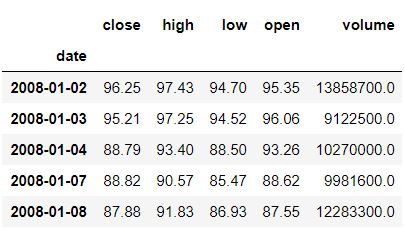Now let us try to simulate the stock prices. For this example, I have taken the Amazon stock data since 2008.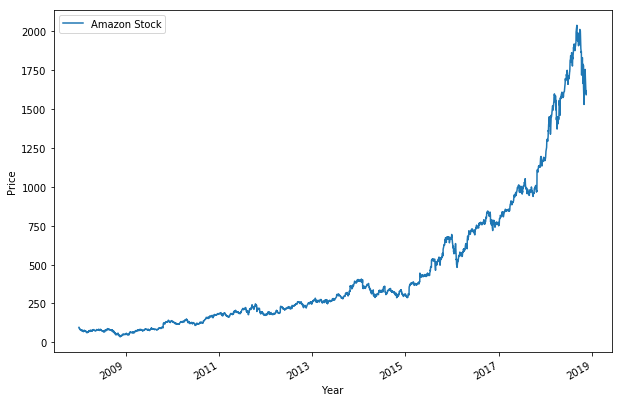Let us simulate the prices for the year 2018, which is almost at a close and compare them with actual stock performance. First, we calculate the sigma and mu parameters from the previous equations.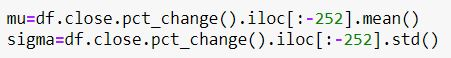Next, we create a dictionary to save all the simulations that we will be making. In this dictionary along with the simulations, we will save the actual stock prices for comparison.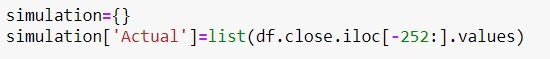Next, to simulate the prices we should begin all the simulations from the same starting price. In this case, we consider the close price of the stock that was one year ago.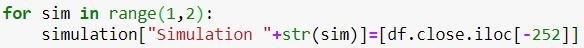Next, we simulate the next days’ close price as per the GBM formula and append it to the simulation data.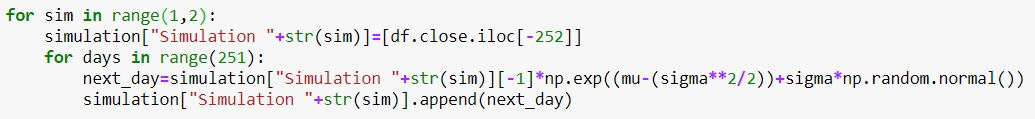Finally, we will plot the simulation and actual prices to visualize it.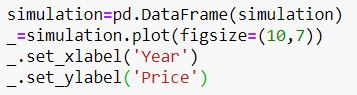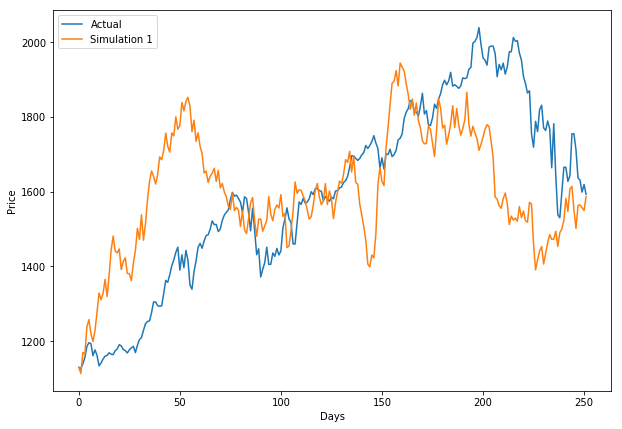### Conclusion

These simulations are very useful when one is interested in finding the VaR or the expected shortfall for a particular stock with a certain degree of confidence. To perform such risk management analysis one should generate as many simulations as possible. In this case, I have simulated the price for 1000 times and the result looks like this.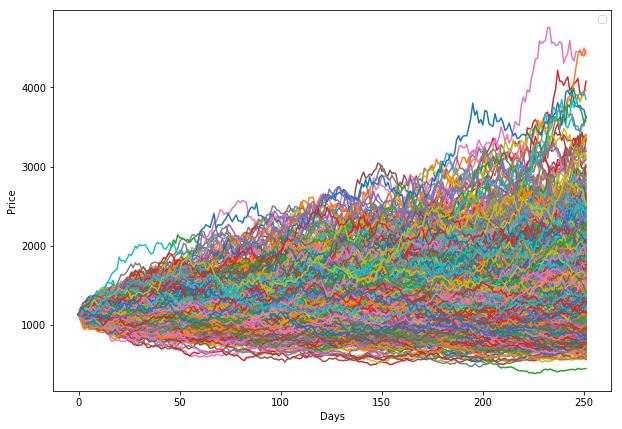Traders have been using a number of stochastic models to identify a winning strategy. You can learn more by taking the seven course bundle which include various quantitative strategies and models.

Disclaimer: All investments and trading in the stock market involve risk. Any decisions to place trades in the financial markets, including trading in stock or options or other financial instruments is a personal decision that should only be made after thorough research, including a personal risk and financial assessment and the engagement of professional assistance to the extent you believe necessary. The trading strategies or related information mentioned in this article is for informational purposes only.